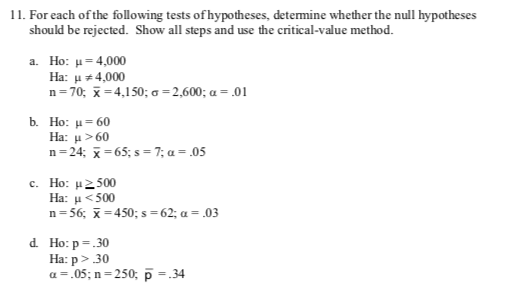# 11. For each ofthe following tests of hypotheses, determine whether the null hypothesesshould be rejected. Show all steps and use the critical-value methoda. Ho: 4,000Ha:u4,000n 70, 4,150; a=2,600; a= .01b Ho: μ 60Ha: μ>60n 24;65;s=7; a = .05c. Ho: 500Ha: 30a.05; n 250; p 34

Question
16 viewshelp_outlineImage Transcriptionclose11. For each ofthe following tests of hypotheses, determine whether the null hypotheses should be rejected. Show all steps and use the critical-value method a. Ho: 4,000 Ha:u4,000 n 70, 4,150; a=2,600; a= .01 b Ho: μ 60 Ha: μ>60 n 24;65;s=7; a = .05 c. Ho: 500 Ha: <500 n 56 -450; s= 62; a = .03 d Ho: p30 Ha: p>30 a.05; n 250; p 34 fullscreen
check_circle

Step 1

d.

Null hypothesis:

H0p=0.30.

Alternative hypothesis:

Hap>0.30.

From the given information, the sample size is, n =250 and the sample proportion is  =0.34.

Test Statistic:

The test statistic is 1.38 and it is calculated below:

Step 2

Critical value:

From the standard normal table. the critical value corresponding with 0.05 and right tail test is 1.645.

Rejection Rule:

If zcal is greater than the critical value, then reject the null hypothesis.

If zcal is lesser than or equal to the critical value, then do not reject the null hypothesis.

Conclusion:

The t...

### Want to see the full answer?

See Solution

#### Want to see this answer and more?

Solutions are written by subject experts who are available 24/7. Questions are typically answered within 1 hour.*

See Solution
*Response times may vary by subject and question.
Tagged in

### Hypothesis Testing